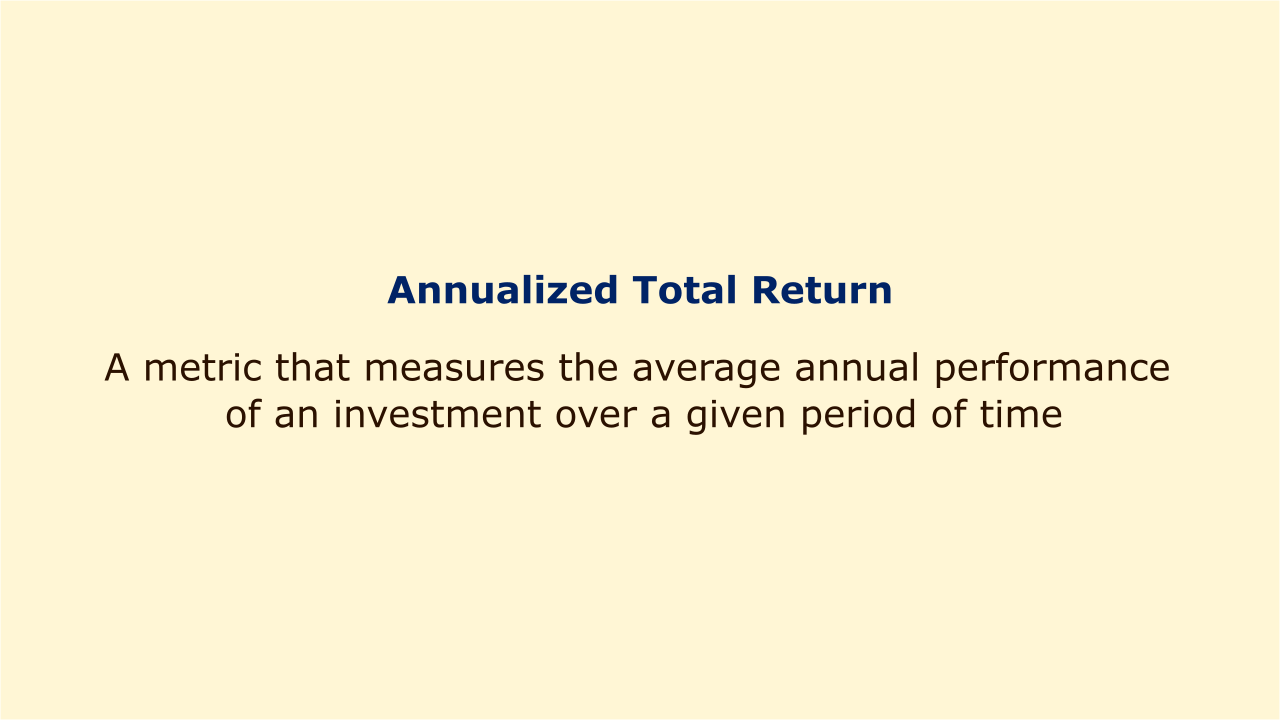# Annualized Total ReturnImage: Moneybestpal.com

### The annualized total return (ATR) indicator calculates the average annual performance of an investment over a specified time period. It is determined as a geometric average, which accounts for the effects of compounding. ATR is often referred to as the Compound Annual Growth Rate (CAGR).

Investors can compare investments with various time horizons and risk profiles using ATR. ATR, however, says nothing about the investments' volatility or price swings.

There are different ways to calculate ATR depending on the available information. Here are some common formulas:

- If the annual returns for each year are given, the formula is:

ATR = ((1 + R1) x (1 + R2) x ... x (1 + Rn))^(1/n) - 1

where R1, R2, ..., Rn are the annual returns for each year and n is the number of years.

- If the cumulative return for a given period is given, the formula is:

ATR = (1 + R)^(365/d) - 1

where R is the cumulative return, 365 is the number of days in a year, and d is the number of days the investment was held.

- If the initial and final values of the investment are given, the formula is:

ATR = (FV / IV)^(1/n) - 1

where FV is the final value of the investment, IV is the initial value of the investment, and n is the number of years.

Here are some examples of how to apply these formulas:
1. Consider a scenario where a shareholder invested \$10 per share in 100 shares of a company for a three-year period. Each year, the stock paid a dividend of \$0.50 per share and had annual gains of 5%, 10%, and 15%. What is the ATR of this investment?

The annual returns for each year are:

R1 = 0.05 + 0.50 / 10 = 0.10

R2 = 0.10 + 0.50 / (10 x 1.05) = 0.1476

R3 = 0.15 + 0.50 / (10 x 1.05 x 1.1476) = 0.1894

The ATR is:

ATR = ((1 + 0.10) x (1 + 0.1476) x (1 + 0.1894))^(1/3) - 1

ATR = 0.1448 or 14.48%

2. Consider a scenario where a bond was purchased for \$1,000 and sold for \$1,050 after 180 days. During this time, the interest on the bond was \$25. What is the ATR of this investment?

The cumulative return for this period is:

R = (1050 + 25 - 1000) / 1000 = 0.075

The ATR is:

ATR = (1 + 0.075)^(365/180) - 1

ATR = 0.1539 or 15.39%

3. Consider a scenario where a buyer paid \$5,000 for a mutual fund and then sold it for \$6,500 after two years. At this time, the fund distributed dividends of \$200. What is the ATR of this investment?

The initial and final values of the investment are:

IV = \$5,000

FV = \$6,500 + \$200 = \$6,700

The ATR is:

ATR = (6700 / 5000)^(1/2) - 1

ATR = 0.1487 or 14.87%
Tags